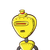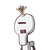# A table top measures 5m 50 cm by 3m 20 cm. Calculate the perimeter of the table top .​

A table top measures 5m 50 cm by 3m 20 cm. Calculate the perimeter of the table top .​

### 2 thoughts on “A table top measures 5m 50 cm by 3m 20 cm. Calculate the perimeter of the table top .​”

1.1740

Step-by-step explanation:

perimeter = 2(l+b)

= 2(550+370)

=2(870)

=1740cm

2.